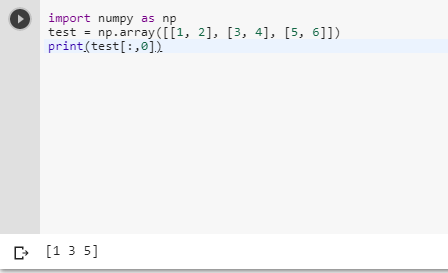2 views
in Python

Suppose I have:

test = numpy.array([[1, 2], [3, 4], [5, 6]])

test[i] gets me ith line of the array (eg [1, 2]). How can I access the ith column? (eg [1, 3, 5]). Also, would this be an expensive operation?

by (106k points)

Python has a wonderful method that is called as slicing this can be used to access the ith column of a NumPy multidimensional array we will use this as follows:-

import numpy as np

test = np.array([[1, 2], [3, 4], [5, 6]])

print(test[:,0])